# A general principle for Hausdorff measure

We introduce a general principle for studying the Hausdorff measure of limsup sets. A consequence of this principle is the well-known Mass Transference Principle of Beresnevich and Velani (2006). https://arxiv.org/pdf/1808.02135.pdf

The main result is as follows.

\begin{theorem}[Hussain-Simmons, 2018]\label{HS:thm}
Fix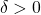, let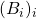be a sequence of open sets in an Ahlfors-regular metric space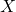, and let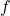be a dimension function such that

(1)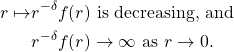Fix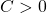, and suppose that the following hypothesis holds:
\begin{itemize}
\item[(*)] For every ball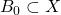and for every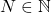, there exists a probability measure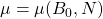with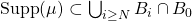, such that for every ball, we have

(2)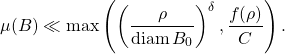\end{itemize}
Then for every ball,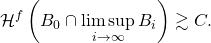In particular, if the hypothesis \text{(*)} holds for all, then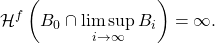\end{theorem}

The condition \eqref{f2} is a natural condition which implies that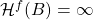. A consequence of this theorem is the celebrated Mass Transference Principle of Beresnevich-Velani (2006).